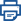Print this pageTitle

# Core TextbookKeiryo Keizai-gaku(Econometrics)

Size

376 pages, A5 format

Language

Japanese

Released

December 10, 2017

ISBN

978-4-88384-264-3

SHINSEI-SHA Co., Ltd.

Book Info

See Book Availability at Library

Japanese Page

view japanese page

This textbook is an introduction to econometrics for undergraduate students using the popular statistical software Stata (version 15). For those who are learning statistics for the first time, Chapter 1 reviews the introductory statistics. In Chapters 2 and 3, simple and multiple linear regression models are introduced, respectively, using the least squares method. These chapters introduce the concepts of the coefficient of determination, the multiple correlation coefficient, interval estimation, partial correlation, and dummy variables. Hypothesis testing for a linear combination of regression coefficients, structural change, and Granger causality are also discussed. Chapter 4 discusses how to address violations of the standard assumptions of the linear regression model. The linear regression model is transformed to analyze panel data with fixed or random effects models in Chapter 5. The Hausman specification test is also discussed. Chapter 6 introduces the logit and probit models for binary data, ordered logit and probit models for ordered data, and multinomial logit and probit models for categorically distributed dependent variable data. Chapter 7 discusses various micro-econometric models, including the Tobit models, sample selection models, Poisson regression models for count data, and Weibull regression models for duration data. In Chapter 8, the simultaneous equation models and seemingly unrelated regression models are discussed. Finally, Chapter 9 introduces the time series models, including the ARMA (autoregressive moving average) and GARCH (generalized autoregressive conditional heteroskedasticity) models.

(Written by OMORI Yasuhiro, Professor, Graduate School of Economics / 2018)

## Related Info

Award:
The Japan Statistical Society 2018
https://www.jss.gr.jp/society/prize/prize_biog2018/

The Japan Statistical Society 2012
https://www.jss.gr.jp/society/prize/